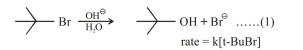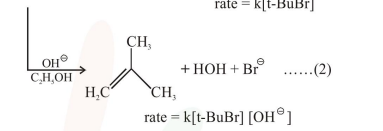# Consider the reaction sequence given below :

Question:

Consider the reaction sequence given below :Which of the following statements is true :

1. Changing the concentration of base will have no effect on reaction (1)

2. Changing the concentration of base will have no effect on reaction (2)

3. Changing the base from $\mathrm{OH}^{\ominus}$ to $^{\ominus} \mathrm{OR}$ will have no effect on reaction (2)

4. Doubling the concentration of base will double the rate of both the reactions.

Correct Option: 1

Solution:

Reaction 1: SN,

Reaction $2: \mathrm{E}_{2}$

$\mathrm{SN}_{1}$ is independent of concentration of nucleophile/base